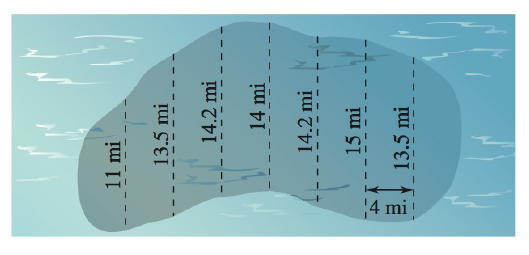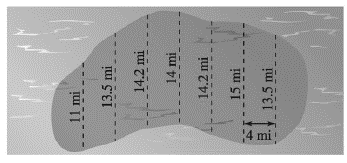Chapter 5.6, Problem 31E### Calculus: An Applied Approach (Min...

10th Edition
Ron Larson
ISBN: 9781305860919

#### Solutions

Chapter
Section### Calculus: An Applied Approach (Min...

10th Edition
Ron Larson
ISBN: 9781305860919
Textbook Problem
1 views

# Surface Area Use the Midpoint Rule to estimate the surface area of the oil spill shown in the figure.To determine

To calculate: The surface area of the oil spill shown in the figure below by application of the midpoint rule,Explanation

Given Information:

The figure showing the oil spill is provided below as,

Formula used:

The midpoint rule states that the midpoint (m) of each interval (a, b) is given by

m=a+b2.

Calculation:

The width of each area is 4 mi.

Calculate the midpoint of each interval as,

[0,11],[11,13.5],[13.5,14.2],[14.2,14],[14,14.2],[14.2,15],[15,13.5],[13.5,]

The midpoint of the interval [0,11] is,

[0,11]=0+112=5.5

The midpoint of the interval [11,13.5] is,

[11,13.5]=11+13.52=12.25

The midpoint of the interval [13.5,14.2] is,

[13.5,14.2]=13.5+14.22=13.85

The midpoint of the interval [14.2,14] is,

[14

### Still sussing out bartleby?

Check out a sample textbook solution.

See a sample solution

#### The Solution to Your Study Problems

Bartleby provides explanations to thousands of textbook problems written by our experts, many with advanced degrees!

Get Started

#### Find more solutions based on key concepts